<< Back to Off-topic Forum .GrayedOutTextBox { color: Gray; }

 Posts 1 - 11 of 11
order of operations is incomplete (math): 7/15/2021 22:18:01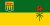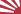goodgame
Level 56
Report
I'm sure you're all familiar with BEDMAS, or something similar, in which you have brackets first, then exponents, then division and multiplication, then addition and subtraction. There's something missing though. 1 - 1 - 1 = ? If you do it as (1 - 1) - 1, you get -1. If you do it as 1 - (1 - 1), then you get 1. So, which is correct? As far as I can tell most people would assume the first option, because in reading you go from left to right, so equations are read the same. I guess that means that we should assume 6 + 4 - 2 - 1 + 3 is (((6 + 4) - 2) - 1) + 3.

A fraction it seems is always considered as a single term, though it's really the same as a division equation. So 2 / 2/2 should be the same as 2 / 2 / 2. But it seems not. Reason being that if you do it the first way, then you simplify 2/2 on the right to 1 first, then figure out 2 / 1 = 2. Whereas, if you do it the second way, then you do 2 / 2 on the left, which is 1, then you do 1 / 2 which is 0.5. Order of operations needs clarification.
order of operations is incomplete (math): 7/16/2021 00:59:31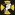Benjamin628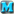Level 60
Report
Completely agree with this take. Math should be done on paper with real symbols. Nobody writes this down:

6 ÷ 2(1 + 2)

Is the answer 1 or 9? Who cares.

6
-------
2(1 + 2)

order of operations is incomplete (math): 7/16/2021 03:28:02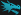Tac(ky)ticalLevel 62
Report
Please. Excuse. My. Dear. Aunt. Sally.

Parantheses, exponents, multiplication, division, addition, subtraction

I don't understand the confusion?
order of operations is incomplete (math): 7/16/2021 03:29:34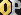StonewallLevel 62
Report
it is assumed you do the operations of the same level left to right. so no there is not more then one result.
order of operations is incomplete (math): 7/16/2021 03:31:49Tac(ky)ticalLevel 62
Report
you can't assume parentheses. also there is big difference between 2 / 2 / 2 and 2 / (2/2) what is 2 divided 2 = 1 divided 2 = .5 and other one is 2 divided 1 = 2
order of operations is incomplete (math): 7/16/2021 14:28:01Anavasi
Level 46
Report
--deleted--

Edited 7/16/2021 14:29:40
order of operations is incomplete (math): 7/16/2021 14:43:32Benjamin628Level 60
Report
Of course I know PEMDAS Tacky. 2 / 2 / 2 is just such a silly way to write out an expression. You should instead write it like this:

https://imgur.com/a/F22LsYN

Top one = 2, bottom one = 0.5. No ambiguity or OOO gymnastics needed.

Edited 7/16/2021 14:44:02
order of operations is incomplete (math): 7/17/2021 01:09:50Photonic Symmetry
Level 60
Report
Expressions shouldn't be written in an ambiguous manner. Simple as that. Even in programming, where you extend PEMDAS for other stuff like bitwise, relational, ternary, and increment operators, one generally tries to make the expression as comprehensible as possible despite there being a specific order in which these operators have precedence.
order of operations is incomplete (math): 7/19/2021 04:10:28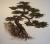BonsaiLevel 63
Report
I would suggest that 2 / 2 / 2 is a poor way to write the expression. The ÷ symbol should be used writing from left to right to represent a simple operation so there is no confusion.

Generally speaking, the symbol "/" here likely implies the simple operation of division that should calculated left to right, so it really should be written 2 ÷ 2 ÷ 2. If you don't wish for this to be the case, then you should rather write 2 ÷ (2 ÷ 2)

When you write something like

6
-------
2(1 + 2)

then the fraction line acts as a grouping symbol. So it is not the same as 6 ÷ 2(1 + 2), which you incorrectly state is unclear.
order of operations is incomplete (math): 7/19/2021 04:25:47l4v.r0vLevel 59
Report
The order of operations is sufficient to make any arithmetic expression unambiguous. If you think it isn't, then you are missing something about the order of operations.

(sidestepping the discussion about whether ambiguous expressions are acceptable)
order of operations is incomplete (math): 7/19/2021 11:43:19Tac(ky)ticalLevel 62
Report
so conclusion: order of operations does NOT need clarification.
 Posts 1 - 11 of 11